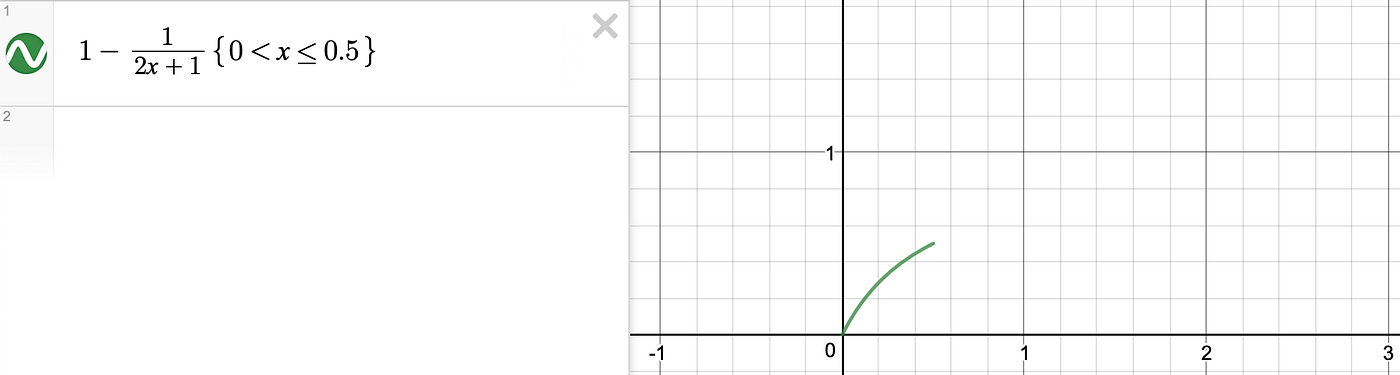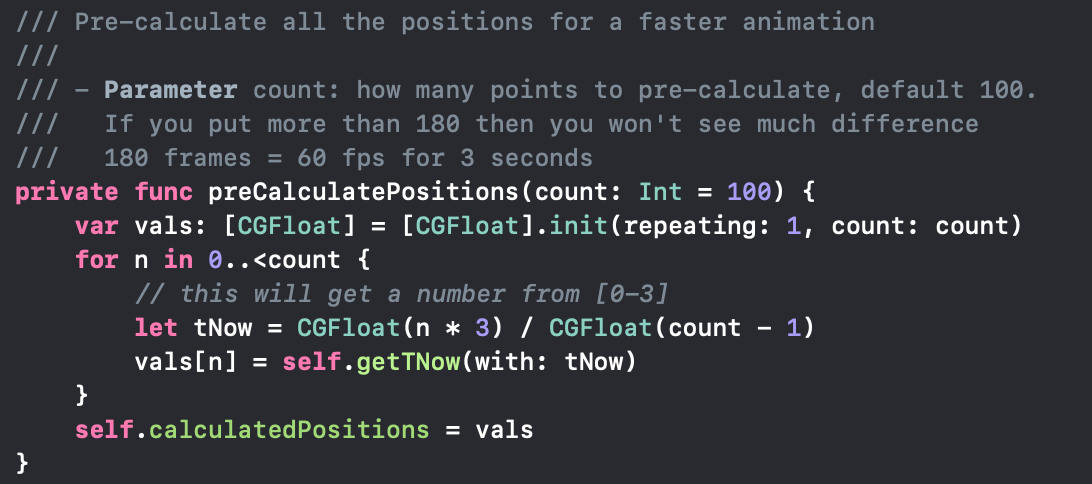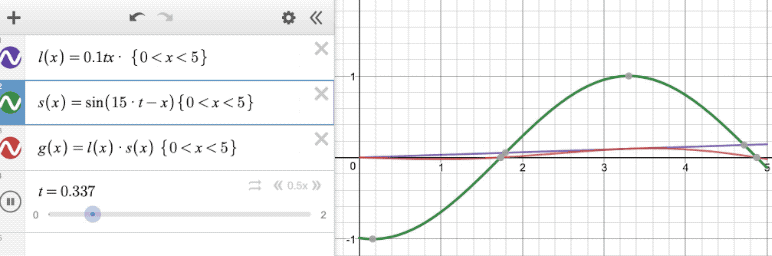# ARKit + SceneKit Geometries Tutorial (Part 2)

If you haven’t already, I highly recommend you check out ARKit + SceneKit Geometries Tutorial (Part 1), there are some core graphics concepts in that which you’ll want to have an understanding of to continue.

So far I’ve shown you examples of how to re-create SCNPlane and SCNBox using primitive types with vertices, normal maps and texture mapping. The next thing I want to discuss is how to make those geometries come alive, in ways that cannot be done by scaling, rotating or translating a Scene Node.

Here’s the GitHub repository to go with this tutorial:

# Animating a single vertex

The first thing we’re going to do is take a single vertex of the cube and have an animation that makes it look like it’s pulling away from the centre of the cube, and then releasing with a wobbling effect as it moves back into place. This GIF below demonstrates the animation:

Click here to see this project on GitHub

The basic idea here is, given a vertex at point `[x,y,z]` I want to pull it to about `[1.5x, 1.5y, 1.5y]`, and then release it back to `[x,y,z]`. The vertex is chosen at random here, but you could add a hitTest to choose the point via a tap instead.

When doing something like this I find it useful to use a graphing calculator, Desmos is really great for that, I’ve been using it for years.

I’ve set up a new class `BoxStretcher`, subclass of `SCNNode`. I’ve also added another class function to `SCNGeometry` to return the vertices and indices for a standard cube with 8 vertices. Another useful function I’ve added to BoxStretch is `updateGeometry()`, which creates the SCNGeometry given the updated vertices and indices and reassigns the node’s geometry whenever a vertex is updated.

The best way I’ve found to animate the geometry updates is with SCNAction.customAction(duration:action:). Whatever you put in the code block is called every frame, which is what we want for most animations.

This is the content of `animateCorner()`, which is updating the vertex and refreshing the geometry every time it’s called:

The above looks a bit complex, so let’s break it down. `elapsedTime` will be a number [0–3], so for the first 0.5 seconds we’ll go through the if, not the else. Using Desmos we can see that the graph for this looks like the following (exchanging time for x):See the tip of the curve reaches [0.5,0.5]

So when `elapsedTime` reaches 0.5, the vertex should be at [x,y,z] * (1 + 0.5), which will be the furthest away that we want it.

Now when we introduce the section after 0.5, if we break it down it’s a sine wave multiplied by an exponential decay. Without the decay it would just continue to wobble forever, but with the decay we get a nice movement of the vertex slowing down and settling back at zero. and when we have zero we have: [x,y,z] * (1 + 0.0); back to our starting point.

Here’s a link to my Desmos graph for this, there’s a point along the y axis that should follow the exact same pattern as our vertex.

Sorry if I lost you there. The point is we want the point to move, in the [x,y,z] direction, but how much based on time since we started? We need a function that takes in time elapsed and outputs how much we should have moved along our curve. This is the same principal used for easing functions, as well as having many other applications.

This method works great, the main difference between baking an animation to a model in another program is that the values of each frame are saved in as part of the file, so the device doesn’t need to calculate the end positions with the calculations I’ve given including sin and exponential. The benefit of baking the animations is it will calculate these values faster, the trade-off is it uses more storage thus memory when loaded with your object. You can find a middle ground by calculating the a number of`tNow` values when the model is first loaded. This is a classic Space–time tradeoff, here’s an example of how to create a lookup table:This saves all the positions into the calculatedPositions array, called anytime after the object is initialised

And then the following `animateCornerPrecalc()` would be called in place of `animateCorner()`

All the above functions are included in the repository.

# Animating multiple vertices

For this section, let’s look once again at a planar surface, rather than a cube.

One use-case for animating a plane would be to have a waving flag. So before coding, I want to get a nice curve for a flag using Desmos.

There are a couple of requirements I’d like for this: to be fixed to the x axis at the origin, but as the point moves away from the edge of the flag they can diverge more from the x axis. I’d want a sinusoidal curve here like before, and a linear increase in the size of the wave as time goes on.Purple shows the linear increase, green the sine wave, and red shows the product of them both, our result

This is essentially a top down view of the plane, I won’t be able to use Desmos to demonstrate how an animation would happen in a third axis very easily, but I’ll provide an example at the end. Click here for a link to the second Desmos graph.

OK so putting the above formula into our xCode project:

I’ve added another static function to SCNGeometry to get the vertices, texture mapping (as a geometry source) and indices for a plane, the function is `PlaneParts(size: CGSize, xyCount: CGSize)` , it takes in two CGSize arguments, the first for the overall width and height, and the second for the number of horizontal and vertical vertices. If the latter is omitted, it will default to 2x2 vertices, the minimum. The more vertices we have, the smoother the flag will look, at the cost of more calculations per frame and more vertices in the scene. This is something you can’t do if importing a geometry from another file, this lets you adjust the vertex count in cases where you know your model is going to be small or far away by doing a quick calculation on creation.

The next class we’re making is FlagNode.

The above function is put in the same place as `animateCorner` was in the previous example. Here, the `waveScale` value is equivalent to `l(x)` in the Desmos GIF above, my purple line. and the right hand side of the multiple that in `newZ` makes up the green curve, resulting in something that follows the product of them both; the red curve. Side note: We could have `for x in 1..<xCount`, as the first one is always zero, but I left it in so that it works the same in anyone’s case.

OK so this flag is ready to create, here it is with 2 x 4 vertices:

`let newNode = FlagNode(  frameSize: CGSize(width: 0.75, height: 0.375),  xyCount: CGSize(width: 4, height: 2),  diffuse: UIImage(named: "union_jack"))`

We need more triangles! We can easily update this to 40x2 vertices. There’s little point updating the vertices from the top to the bottom, as they will still fall in a straight line so won’t have any effect on the look of the flag, just add more computation to our scene for no reason.

This one looks much better, but see towards the end we can still see the individual polygons when close, let’s up it once more.

`let newNode = FlagNode(  frameSize: CGSize(width: 0.75, height: 0.375),  xyCount: CGSize(width: 100, height: 2),  diffuse: UIImage(named: "union_jack"))`

Perfect, we can no longer see the individual polygons on this flag and so this is a great result for me! The frame rate on this GIF isn’t great by the way, clone the repo to see it at 60fps.

To finish, I’m going to update the animation shown above to depend on the y axis as well as the x for the waves.

The main difference here is that the total distance (Manhattan distance) is calculated in the second for loop, while the distance along the X axis is calculated the same as before. The left edge will still be locked in place but towards the right edge there will be another wave interfering in the Y axis.

For this we have increased the number of vertices in the y axis of the plane to give it a really fluid look, unlike the first flag GIF above.

From here you can create a whole world of animations right within xCode without first learning how to use a 3D graphics program like Blender, 3ds Max etc. You can also make your objects optimised for your scene by creating classes similar to those created through this tutorial.

Here’s one last flag that I made by accident, and left the code on GitHub for you to play with but I basically made the wavelength of the sine curve way too short!

In a future tutorial I’d like to talk about shaders which, as Alberto Taiuti pointed out, would be a preferred alternative to these animations on objects like the waving flag. There’s much much more you can do with shaders, and the code snipped below shows you one way of making a flag with a waving animation using just a geometry shader in SceneKit using a String:

However, it requires knowledge of another C-style language as you can see, so deserves its entire own article to fully explain and understand. (Coming Soon!)

Thanks for reading! I hope you’ve learned something from this tutorial. I hope to make more of these posts soon.

Find me on Twitter or LinkedIn if anything’s unclear or have any suggestions for this post or thoughts for future posts; also check out my GitHub for other public projects I’m working on.

--

--